### Home > CALC > Chapter 4 > Lesson 4.2.3 > Problem4-73s

4-73s.
1. Katherine is babysitting her calculus teacher’s daughters Natalie, Morgan, and Lydia. Katherine’s pay depends on the rate function g(x) = 4x + 3 where x is the number of hours spent babysitting and g(x) is the rate of pay in dollars per hour. Her calculus teacher is strict about sending text messages while babysitting and adds a hole to the pay rate function for every instance a message is sent. For example, if Katherine sends a text message two hours into babysitting her new rate function will be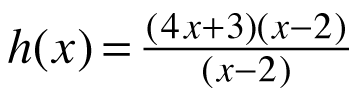.

1. Assume Katherine worked for 0 ≤ x ≤ 3 hours. Use your calculator’s graphical integration feature to determine the total amount of money Katherine earns for g(x) = 4x + 3 and.

2. Should Katherine worry about losing pay when she sends a text message? Explain your reasoning.

3. How can this discontinuous situation be represented using integrals?

4. What would happen if Katherine sent text messages the entire time she was babysitting?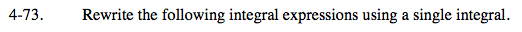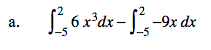Notice that the bounds are the same but the integrands are different. What this means is that, on the interval x = −5 through x = 2, we are subtracting the area under y = −9x from the area under y = 6x3.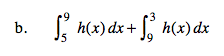Since both integrands are the same, h(x), notice the bounds. The first integral has bounds that move forward, starting at x = 5 and
ending at x = 9. While the second integral has bounds that move backwards, from x = 9 to x = 3. What area remains?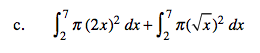Refer to the hint in part (a). Remember that you can factor out π.Notice that both the integrands and the bouds are different. The only thing that these integrals have in common is the distance between the bounds: 4 − 1 = 9 − 6. We can shift the first integral 5 units to the right to match the second integral, or vice versa.

You will not change the bounds, be sure to shift the function accordingly:
f(x − 5) will shift the function 5 units to the right.
f(x + 5) will shift the function 5 units to the left.